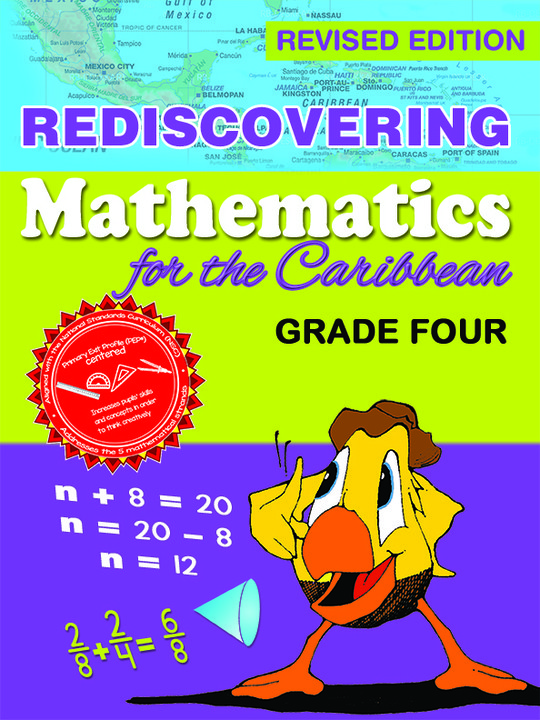\$9.99

# Rediscovering Mathematics for the Caribbean: Grade 4 (Revised Edition)

US\$ 9.99
The publisher has enabled DRM protection, which means that you need to use the BookFusion iOS, Android or Web app to read this eBook. This eBook cannot be used outside of the BookFusion platform.
Book Description

Rediscovering Mathematics for the Caribbean: Grade Four, is the sixth of a comprehensive series of eight textbooks which covers the requirements of the Mathematics syllabuses for primary schools in the Caribbean. The text provides a firm foundation in Mathematics for primary school pupils utilizing a learner-centred approach.

Some Key Features:

Basic numeracy skills are emphasized and topics are carefully introduced.
Numerals, exercises and illustrations provide pupils with a lot of practice, thereby enhancing their understanding of mathematical concepts.
Concepts are expressed in simple, clear and easy to understand language that is appropriate to the grade level and subject.
Meaningful connections are made to real life situations.

Other features include activities that provide opportunities for students to engage in critical thinking and problem solving, and students are required to perform tasks that extend and reinforce knowledge and skills learnt. The language used throughout the series is simple and straightforward, and pupils and teachers should find the learning and teaching experience fun and quite enjoyable.

• Cover
• Acknowledgement
• Title Page
• Preface
• Contents
• Number
• Sets
• Kinds of Numbers
• Place Value
• Working with Seven (7) Digit Numbers
• Writing Numbers in Words
• Value
• Expanded Form
• Expanding Using Words
• Writing Numbers in Standard Form
• Problem Solving
• Subtraction
• Subtraction of Whole Numbers
• Rules of Subtraction
• Bar Modelling
• Using the Bar Model to Solve Subtraction Problems
• Using the Bar Model Method with Money
• Problem Solving
• Mixed Operations
• Multiplication
• Multiplication of Whole Numbers by One-Digit
• Multiplication by Two-Digit
• Problem Solving
• Multiplying by Ten
• Counting in Tens
• Division
• Terms in Division
• Long Division
• Steps to Take in Long Division
• Divide the Hundreds
• Division by Two-Digit Numbers
• Bar Modelling
• The Comparision Model (Multiplication and Division)
• Open Sentences
• Order of Operations
• Rounding Numbers
• The Calculator
• Fractions
• Equivalent Fractions
• Using Diagrams to Rename Fractions
• Using Multiplication to Rename Fractions
• Using Division to Rename Fractions
• Reducing Fractions
• Comparing Fractions
• Comparing Fractions with Like Denominators
• Comparing Fractions with Unlike Denominators
• Types of Common Fractions
• Improper Fractions
• Mixed Numbers/Mixed Fractions
• Changing Mixed Numbers to Improper Fractions
• Addition of Fractions with Like Denominators
• Adding Like Fractions with Like Remainder
• Subtraction of Like Fractions with Whole Numbers
• Subtracting Fractions with Whole Numbers
• Adding Mixed Numbers (Like Denominators)
• Subtraction of Mixed Numbers (Like Denominators)
• Subtrating Fractions (Unlike Denominators)
• Adding Mixed Numbers (Unlike Denominators)
• Subtracting Mixed Numbers (Unlike Denominators)
• Mixed Operations
• Fractions
• Mulitiplying Fractions by Whole Numbers
• Multiplying Mixed Numbers
• Diving Fractions
• Reciprocal
• Dividing Fractions and Wholes
• Dividing Fractions by Fractions
• Dividing Mixed Numbers
• Mixed Operations
• Multiplication and Division
• Factors
• Common Factors
• Highest Common Factors (H.C.F.)
• Prime Factors
• Prime Factorization
• Multiples
• Least/Lowest Common Multiple (L.C.M.)
• Decimals
• What is a Decimal?
• Place Value
• Comparing Decimals
• Subtraction of Decimals
• Multiplying Decimals
• Multiplying by Powers of Ten
• Dividing By Powers of Ten
• Dividing Decimals by Whole Numbers
• Dividing Decimals by Decimals
• Percentage
• Expressing Percentage as a Decimal
• Expressing Decimals as Percentage
• Changing Fractions to Percentage
• Expressing Percentage as a Fraction
• Extended Thinking
• Measurement
• The Metric System of Measurement
• The Metre
• Addition and Subtraction of Units
• Simple Scale Drawing
• Mapping
• The Gram
• Types of Scales
• Units of Mass
• The Litre
• Units of Capacity
• Time
• Telling Time Past the Hour
• Telling Time to the Hour
• Using a.m. and p.m. times
• Units of Time
• Problem Solving
• Temperature
• Money
• Add the Following Notes and Coins
• Subtraction of Money
• Multiplication of Money
• Division of Money
• Extended Thinking
• Geometry
• Geometric Ideas
• Point, Line Segment, Line, ray, Plane
• Kinds of Lines
• Angles
• Measuring Angles
• Kinds of Angles
• Drawing Angles
• Notebook
• Polygons
• What is a Polygon?
• Types of Polygons
• Triangles
• Types of Triangles
• Calculating Angles in Triangles
• Perimeters
• Triangle
• Problem Solving
• Rectangle
• Probelm Solving
• Square
• Perimeter of Irregular and Compound Shapes
• Area
• Areas of Squares and Rectangles
• Area of Rectangle
• Area of Squares
• Area of Irregular Shape
• Area of Compound Shapes
• Congruency
• Non-Congruent Shapes
• Geometrical Solids
• Lines of Symmetry
• Finding the Line of Symmetry with a Mirror
• The Circle
• Algebra
• Number Phrases
• Substitution
• Writing Equations
• Solving Equations
• Patterning and Algebra
• Describing Patterns
• Creating Number Patterns
• Creating and Equation to Calculate Any Team
• Data Handling, Statistics and Probability
• Statistics
• Data Handling
• Graphs
• Pictographs
• Bar Graphs
• Line Graphs
• Circle Graph/Pie Graph or Pie Chart
• Interpreting Data
• Mean
• Median
• Mode
• Range
• Probability
• Extended Thinking
• Assessment
• Assessment One
• Assessment Two
• Assessment Three
• Glossary
• Index
You May Also Like